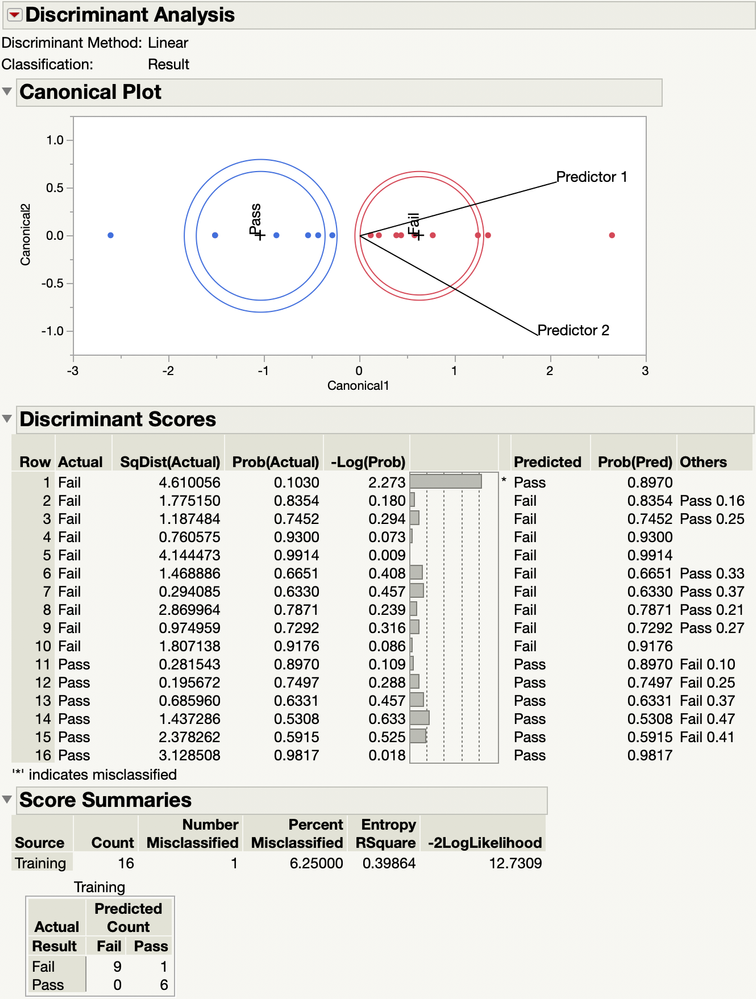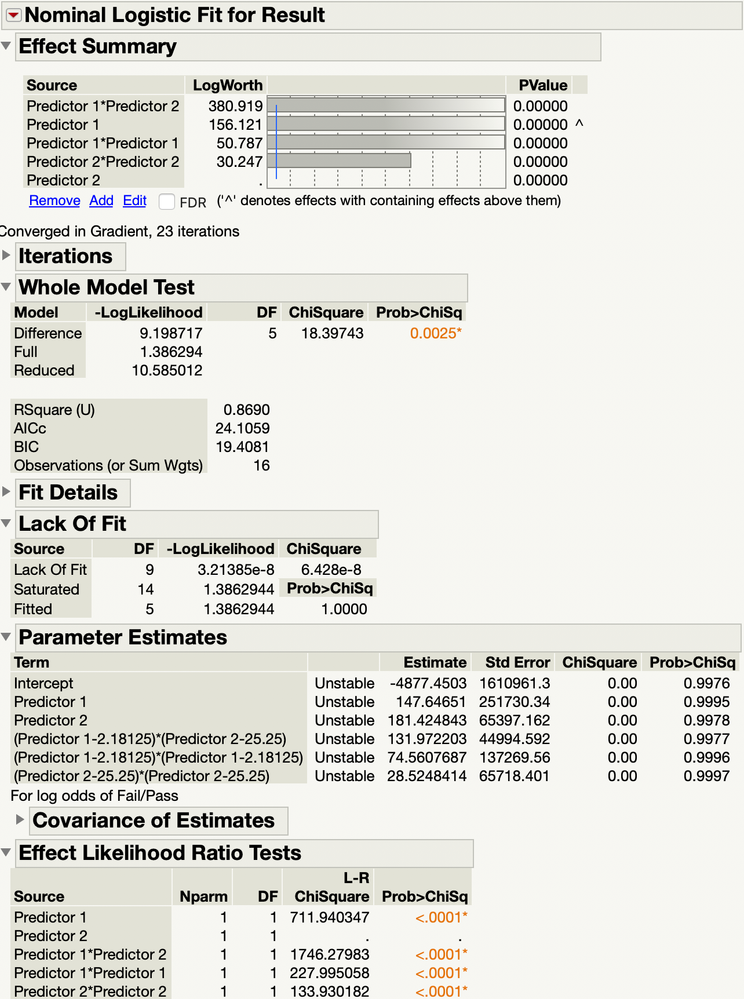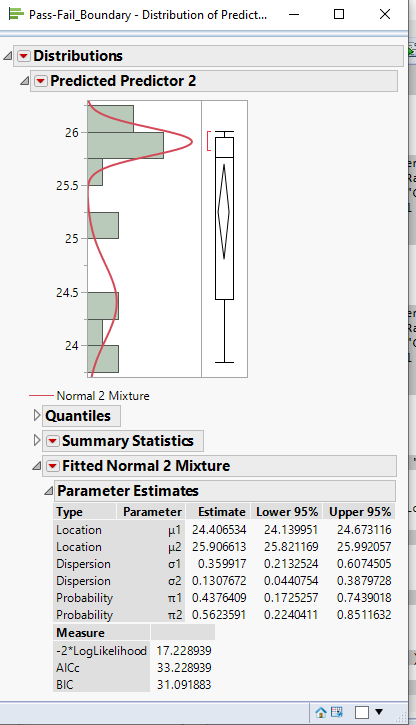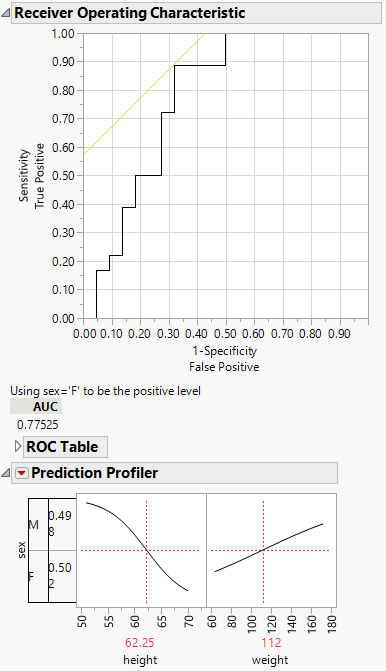The World Statistics Day celebration continues here in the Community. We all need reliable data for sound decision making. Do you have a data source that you trust most? Head over to Discussions to tell us about it.
Choose Language Hide Translation Bar
Highlighted

## Boundary equation, a la phase boundary

Hi.

I have some data where each set appears to have a linear boundary between "pass" and "no pass" results from 2 factor tests. Is there a way to calculate the boundary equation between the two populations of results?

Thank you for advise. Example of a data set is attached.

2 ACCEPTED SOLUTIONS

Accepted Solutions
Highlighted

## Re: Boundary equation, a la phase boundary

This example is a case of classification. There are many techniques for this goal. One, in particular, that might satisfy your need for the 'boundary' is the linear discriminant function. Here is the result applied to your data:This classification is quite good for the binary response with one 1 in 16 misclassification.

Select Analyze > Multivariate Methods > Discriminant. Select the predictors and click Y, Covariates. Select the response and click X, Categories. (Yes, this way seems the opposite of the usual meaning of the X and Y analysis roles.) Click OK.

I suggest that you see Help > Books > Multivariate Methods and the chapter about the Discriminant platform for more information.

Learn it once, use it forever!
Highlighted

## Re: Boundary equation, a la phase boundary

Another popular classification method is binary logistic regression. Here is the result of such an analysis of your data:The response is the logit( Result ) versus the linear predictor (linear combination), so the interpretation is more familiar perhaps than the discriminant function.

See the chapter about the Nominal Logistic platform in Help > Books > Fitting Linear Models.

Recursive partitioning also provides classification but because of the strong linear relationships in this case, it would require very many splits. Such a huge tree would be more difficult to interpret.

Learn it once, use it forever!
6 REPLIES 6
Highlighted

## Re: Boundary equation, a la phase boundary

There might be a better way......Hopefully another community member will speak up.....but what you might want to try, is to run the regression, and save the predicteds, and then using the Distribution Platform, Fit a Normal 2 Mixture distribution.  It will give you the Mean and Sigma of the 2 distributions, and from there you should be able to estimate a division pointJim
Highlighted

## Re: Boundary equation, a la phase boundary

This example is a case of classification. There are many techniques for this goal. One, in particular, that might satisfy your need for the 'boundary' is the linear discriminant function. Here is the result applied to your data:This classification is quite good for the binary response with one 1 in 16 misclassification.

Select Analyze > Multivariate Methods > Discriminant. Select the predictors and click Y, Covariates. Select the response and click X, Categories. (Yes, this way seems the opposite of the usual meaning of the X and Y analysis roles.) Click OK.

I suggest that you see Help > Books > Multivariate Methods and the chapter about the Discriminant platform for more information.

Learn it once, use it forever!
Highlighted

## Re: Boundary equation, a la phase boundary

Another popular classification method is binary logistic regression. Here is the result of such an analysis of your data:The response is the logit( Result ) versus the linear predictor (linear combination), so the interpretation is more familiar perhaps than the discriminant function.

See the chapter about the Nominal Logistic platform in Help > Books > Fitting Linear Models.

Recursive partitioning also provides classification but because of the strong linear relationships in this case, it would require very many splits. Such a huge tree would be more difficult to interpret.

Learn it once, use it forever!
Highlighted

## Re: Boundary equation, a la phase boundary

Thank you all! Building upon the logistic regression solution, I have noticed that Lin[Fail] function is positive for [Fail] category and negative for [Pass] category. So, it is intuitive to suggest that Lin[Fail] is zero on the boundary between the predicted [Pass] and [Fail] categories. Since the formula for Lin[Fail] is an output from the model, equating Lin[Fail] to zero in this formula gives the boundary equation I am after.

Highlighted

## Re: Boundary equation, a la phase boundary

That is correct. The Lin[Fail] stores the linear predictor from the fitted model. It represents the Logit. When the logit is zero, the odds are 1, so the probability of Fail and not Fail are equal.

Learn it once, use it forever!
Highlighted

## Re: Boundary equation, a la phase boundary

There are visualizations for the logistic model to help with the interpretation and exploitation of the model. I used the Big Class data set in the Sample Data folder to fit a model Logit( sex ) F( height, weight). Here are two of the plots:I set the height and weight values to (nearly) achieve zero output. You can see that the probability of the outcome is essentially 0.5 either way.

Learn it once, use it forever!
Article Labels

There are no labels assigned to this post.